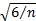/

### Interactively run this function

 Parameter Name Input An input expression? k n

 Calculation description Time-stamp calculation?

### Function Description

Returns the (large sample) confidence point for the (sample) skew of a series of observations, given the null hypothesis that the observations are coming from a normal distribution. See e.g. Press et al. (2007). Is calculated from the quantile point of a normal distribution with standard deviationwhereis the (effective) number of observations.

Contents | Prev | Next